# AP Chemistry : Reaction Types

## Example Questions

### Example Question #2 : Titrations And Indicators

Where does the flattest region of a titration curve of the titration of a weak acid with a strong base occur?

At a pH greater than 7

At the pKa of the acid

At the pKb of the base

At a pH of 7

At the pKa of the acid

Explanation:

In this question, titration curve would graph the pH of acid solution versus the amount of base added. Since the base is strong and the acid is weak, we can conclude that the pH will be slightly greater than 7 at the equivalence point. The equivalence point is found in the steepest region of the curve.

The half-equivalence point is the flattest region of the titration curve and is most resistant to changes in pH. This corresponds to the pKa of the acid. Within this region, adding base (changing the x-value) results in very little deviation in the pH (the y-value). This region is also the buffer region for the given acid.

### Example Question #1 : Titrations And Indicators

You have a solution of weak base with unknown concentration. What would be a good acid with which to titrate the weakly alkaline solution, in order to determine its concentration?

Citric acid

Hydrofluoric acid

Nitric acid

Sodium hydroxide

Stearic acid

Nitric acid

Explanation:

When you titrate a weak base, you want to titrate it with a strong acid. Hydrofluoric acid, citric acid, and stearic acid are all weak acids, and sodium hydroxide is a strong base. The best choice it nitric acid, a strong acid.

### Example Question #1 : Titrations And Indicators

You have a 500mL solution of a monoprotic acid with unknown concentration. You titrate it to completion with 36mL of 0.4M NaOH solution. What is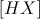?

There is not enough information to solve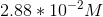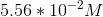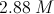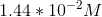Explanation:

If we are working with a monoprotic acid, our chemical equation is: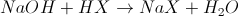Now we will calculate the moles of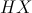in our solution: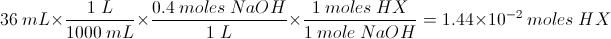Now we will determine the concentration from the amount of moles and the volume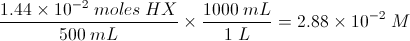### Example Question #1 : Titrations And Indicators

0.458g of an unknown diprotic acid is dissolved in water. It is then titrated with 21.5ml of a 0.500M NaOH solution to reach the second equivalence point. Determine the molecular weight of this unknown acid.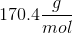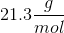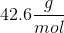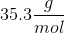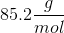Explanation:

To find the molecular weight, we must determine the number of moles that correspond to the 0.458g sample. At the equivalence point, the moles of hydronium ions will equal the moles of hydroxide ions.

We need to use the molarity and volume of the NaOH that was added to find the number of moles of base added. This will tell us the moles of hydroxide ions.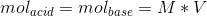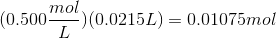Now, since we are working with a diprotic acid, two moles of base would be required for every one mole of the acid. The moles of acid would be: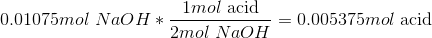Now that we know the moles of acid in the sample, we can use the given sample mass to find the molecular weight.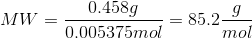### Example Question #131 : Reactions And Equilibrium

Litmus paper may be used to estimate the pH of a solution colorimetrically. The specific color change observed is dependent on the pH of the solution in which it is submerged.

Based on the following figure, what color would you expect a piece of litmus paper to turn when exposed to a sodium hydroxide solution made by adding 0.004g of anhydrous sodium hydroxide (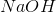) to 1L of water?

Assume the solution's volume is unchanged by the addition of the solid.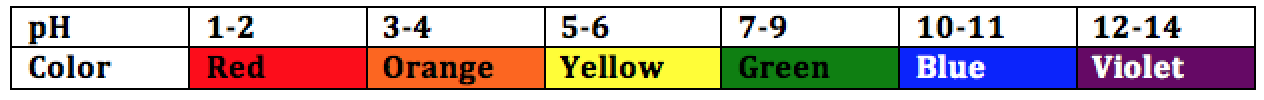Orange

Yellow

Green

Blue

Blue

Explanation:

To determine the predicted color change, the pH of the solution must be determined.

The molar mass ofis approximate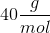.is a strong base and dissociate completely in a 1:1 ratio into its counterions (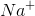and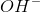).

The concentration ofdetermines the pH of the solution and may be found by calculating the number of moles ofdissolved in solution and dividing by the total volume: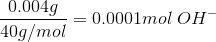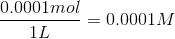Next, we find the pOH of the solution, from which the pH may be easily determined: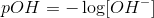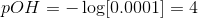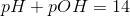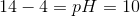According to the given figure, a pH of 10 corresponds to a color change to blue.

### Example Question #81 : Reaction Types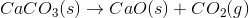The reaction above could be classified as __________.

decomposition

oxidation-reduction

decomposition and oxidation-reduction

synthesis

single displacement

decomposition

Explanation:

When one molecule is converted into two or more smaller molecules, the reaction is considered a decomposition reaction. In this example, none of the elements under go a change in oxidation number, so this is not an oxidation-reduction reaction. (Calcium is always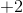, oxygen is always, and carbon remains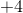throughout the reaction.)

### Example Question #1 : Addition And Decomposition Reactions

Which of the following represents a combustion reaction?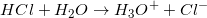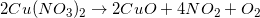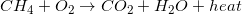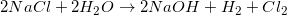Explanation:

Combustion reactions always produce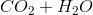and excess heat. Depending on the molecule that is oxidized by oxygen, which in this case was methane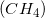, the ratios between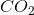and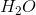can vary.

### Example Question #1 : Balancing Chemical Equations

What is the chemical formula of the salt formed when a chemist mixes solvated Potassium and Arsenic ions in solution?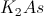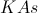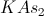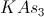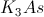Explanation:

Potassium is a Group I element, so to get to a filled valence shell, it will lost one electron, yielding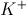.

Arsenic is a Group 5 element, so it needs to gain three electrons to obtain a filled valence shell, yielding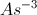.

In order to balance out the charges, the resultant salt will be.

### Example Question #82 : Reaction Types

What is the net ionic equation for the ion exchange reaction between ferrous sulfate and calcium iodide? Assume all compounds are soluble.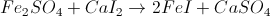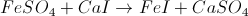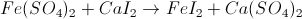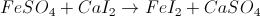Explanation:

First, we must know what ferrous sulfate is. Ferrous refers to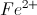, and sulfate has the formula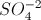. When we combine the two together we get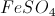.

Calcium is a divatent cation and iodide is a monovalent anion, so their salt is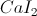. The ion exchange reaction is then:### Example Question #2 : Balancing And Predicting Reactions

Select the net ionic equation from this molecular reaction: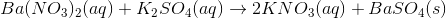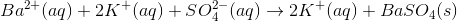None of the other choices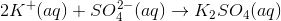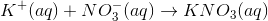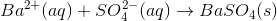Explanation:

The net ionic equation is derived by removing all spectator ions from the total ionic equation (in which all ions are listed). To put it another way, the net ionic equation involves only the ions that participate in a reaction which, in this case, is the precipitation of barium sulfate.

Begin by writing all aqueous compounds in their dissociated (ionic) forms.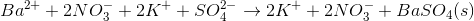Cancel out any ions that appear in equal quantities on both sides of the equation. In this case, we can cancel the nitrate and potassium ions.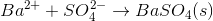This is our net ionic equation.

### All AP Chemistry Resources